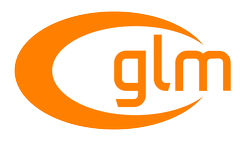0.9.9 API documentation
GLM_GTC_integer

Include <glm/gtc/integer.hpp> to use the features of this extension. More...

Functions

template<length_t L, typename T , qualifier Q>
GLM_FUNC_DECL vec< L, int, Q > iround (vec< L, T, Q > const &x)
Returns a value equal to the nearest integer to x. More...

template<typename genIUType >
GLM_FUNC_DECL genIUType log2 (genIUType x)
Returns the log2 of x for integer values. More...

template<length_t L, typename T , qualifier Q>
GLM_FUNC_DECL vec< L, uint, Q > uround (vec< L, T, Q > const &x)
Returns a value equal to the nearest integer to x. More...

Detailed Description

Include <glm/gtc/integer.hpp> to use the features of this extension.

Allow to perform bit operations on integer values

Function Documentation

 GLM_FUNC_DECL vec glm::iround ( vec< L, T, Q > const & x )

Returns a value equal to the nearest integer to x.

The fraction 0.5 will round in a direction chosen by the implementation, presumably the direction that is fastest.

Parameters
 x The values of the argument must be greater or equal to zero.
Template Parameters
 T floating point scalar types.
GLSL round man page
GLM_GTC_integer
 GLM_FUNC_DECL genIUType glm::log2 ( genIUType x )

Returns the log2 of x for integer values.

Usefull to compute mipmap count from the texture size.

GLM_GTC_integer
 GLM_FUNC_DECL vec glm::uround ( vec< L, T, Q > const & x )

Returns a value equal to the nearest integer to x.

The fraction 0.5 will round in a direction chosen by the implementation, presumably the direction that is fastest.

Parameters
 x The values of the argument must be greater or equal to zero.
Template Parameters
 T floating point scalar types.